Author: Oscar Cronquist Article last updated on February 17, 2023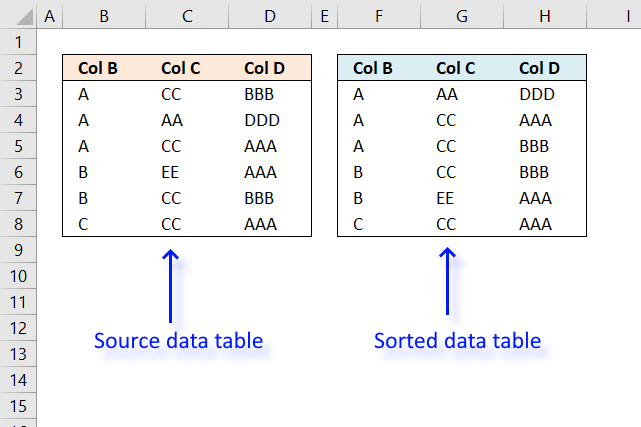I will in this article demonstrate three different techniques to sort a data set in Excel. The first method sorts a data set using array formulas and explain in detail how the formulas work. Why would you want to sort a table using array formulas when Excel has tools built-in that works great? Formulas are dynamic and change instantly when new values are added.

If you have built an interactive worksheet or Dashboard you may have form controls, active-x controls, drop-down Lists or something else connected to your data. The Excel user may not be happy to manually sort values using the features Excel provides.

It may be tedious and time-consuming to sort values each time conditions change or data is added even if built-in tools is used.

The sorted table to the right is created with an array formula using the data in the table to the left. It is sorted by the first column and then by the second column and lastly the third column. The order is from A to Z.

The second techniques is to manually sort the data set using the built in tools Excel provides.

The last technique I will demonstrate is to use a macro that sorts values based on certain conditions like if a value is changed or a worksheet is activated etc, it is event code that makes this possible. They are not placed on the same module as regular macros but in worksheet or workbook modules which I will also describe in detail in this article.

### Sort using array formulas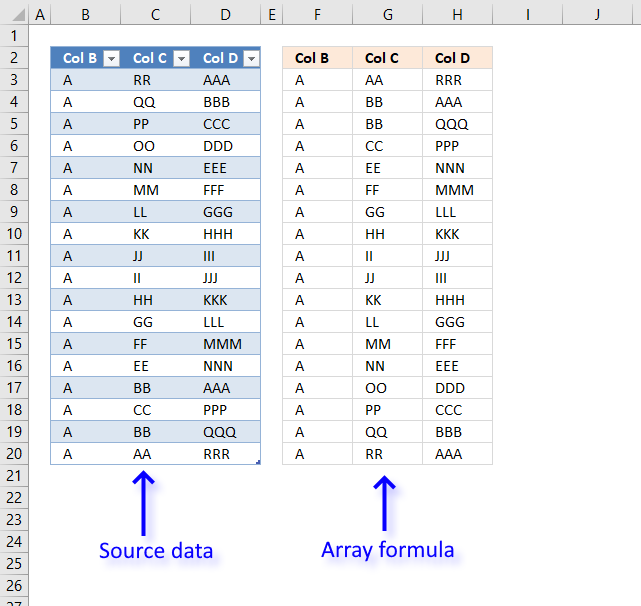The following formula adapts to as many as there are rows in the Excel Table making this feasible for any size of data set. If you have huge amounts of data I recommend using the VBA macro instead, it will be much faster.

Update! Excel 365 dynamic array formula in cell F3:

=SORTBY(Table1,Table1[Col B],1,Table1[Col C],1,Table1[col D],1)

The SORTBY function sorts a cell range or array based on values in a corresponding range or array.

Function syntax: SORTBY(array, by_array1, [sort_order1], [by_array2, sort_order2],…)

Array formula in cell F3:

=INDEX(\$B\$3:\$D\$20, MATCH(SMALL(COUNTIF(\$B\$3:\$B\$20, "<"&\$B\$3:\$B\$20)+COUNTIF(\$C\$3:\$C\$20, "<"&\$C\$3:\$C\$20)/(10^CEILING(LOG10(ROWS(Table1[Col B])), 1))+COUNTIF(\$D\$3:\$D\$20, "<"&\$D\$3:\$D\$20)/(10^CEILING(LOG10(ROWS(Table1[Col B])), 1))^2, ROW(B1)), COUNTIF(\$B\$3:\$B\$20, "<"&\$B\$3:\$B\$20)+COUNTIF(\$C\$3:\$C\$20, "<"&\$C\$3:\$C\$20)/(10^CEILING(LOG10(ROWS(Table1[Col B])), 1))+COUNTIF(\$D\$3:\$D\$20, "<"&\$D\$3:\$D\$20)/(10^CEILING(LOG10(ROWS(Table1[Col B])), 1))^2, 0), COLUMN(A1))

### How to enter an array formula

1. Copy array formula.
2. Select cell F3.
3. Press with left mouse button on in formula bar.4. Paste array formula to formula bar.
5. Press and hold CTRL + SHIFT.
6. Press Enter.

### How to copy array formula

1. Select cell F3.
2. Copy cell (Ctrl +c).
3. Select cell range G:3:H3.
4. Paste (Ctrl + v).
5. Select cell range F3:H3.
6. Copy (Ctrl + c).
7. Select cell range F4:H8.
8. Paste (Ctrl + v).

### Explaining array formula in cell F3

#### Step 1 - Sort Col B from A to Z using the COUNTIF function

The COUNTIF function counts cells based on a condition, however, using a different approach with a less than sign you can create an array containing numbers representing the sort order.

The relative position of each number in the array corresponds to the same value in cell range \$B\$3:\$B\$8.

COUNTIF(\$B\$3:\$B\$20, "<"&\$B\$3:\$B\$20)

becomes

COUNTIF({"A"; "A"; "A"; "A"; "A"; "A"; "A"; "A"; "A"; "A"; "A"; "A"; "A"; "A"; "A"; "A"; "A"; "A"}, {"<A";"<A";"<A";"<A";"<A";"<A";"<A";"<A";"<A";"<A";"<A";"<A";"<A";"<A";"<A";"<A";"<A";"<A"})

and returns

{0; 0; 0; 0; 0; 0; 0; 0; 0; 0; 0; 0; 0; 0; 0; 0; 0; 0}.

#### Step 2 - Sort Col C from A to Z with COUNTIF function

This part of the formula also creates an array containing numbers representing the sort order of each item in column C, however, it also divides the numbers with 10, 100 or 1000 etc based on the number of rows in the data set.

The ROWS function returns the number of rows in a cell range or a structured reference meaning a cell reference pointing to an Excel Table.

COUNTIF(\$C\$3:\$C\$20, "<"&\$C\$3:\$C\$20)/(10^CEILING(LOG10(ROWS(Table1[Col B])), 1))

becomes

COUNTIF(\$C\$3:\$C\$20, "<"&\$C\$3:\$C\$20)/(10^CEILING(LOG10(18), 1))

The next steps rounds the number to the nearest power of 10. Example, 5 returns 10. 99 returns 100. 101 returns 1000 and so on.

The LOG10 function calculates the logarithm of a number using the base 10.

COUNTIF(\$C\$3:\$C\$20, "<"&\$C\$3:\$C\$20)/(10^CEILING(LOG10(18), 1))

becomes

COUNTIF(\$C\$3:\$C\$20, "<"&\$C\$3:\$C\$20)/(10^CEILING(1.25527250510331, 1))

The CEILING function rounds a number up to its nearest multiple.

COUNTIF(\$C\$3:\$C\$20, "<"&\$C\$3:\$C\$20)/(10^CEILING(1.25527250510331, 1))

becomes

COUNTIF(\$C\$3:\$C\$20, "<"&\$C\$3:\$C\$20)/(10^2)

The ^ character works just like the POWER function, it calculates a number raised to a power.

COUNTIF(\$C\$3:\$C\$20, "<"&\$C\$3:\$C\$20)/(10^2)

becomes

COUNTIF(\$C\$3:\$C\$20, "<"&\$C\$3:\$C\$20)/100

and returns

{0.17; 0.16; 0.15; 0.14; 0.13; 0.12; 0.11; 0.1; 0.09; 0.08; 0.07; 0.06; 0.05; 0.04; 0.01; 0.03; 0.01; 0}.

#### Step 3 - Sort Col D from A to Z with COUNTIF function

Column D is the third and last column in the data set.

COUNTIF(\$D\$3:\$D\$20, "<"&\$D\$3:\$D\$20)/(10^CEILING(LOG10(ROWS(Table1[Col B])), 1))^2

becomes

COUNTIF(\$D\$3:\$D\$20, "<"&\$D\$3:\$D\$20)/100^2

becomes

COUNTIF(\$D\$3:\$D\$20, "<"&\$D\$3:\$D\$20)/10000

and returns

{0; 0.0002; 0.0003; 0.0004; 0.0005; 0.0006; 0.0007; 0.0008; 0.0009; 0.001; 0.0011; 0.0012; 0.0013; 0.0014; 0; 0.0015; 0.0016; 0.0017}.

#### Step 4 - Add all arrays

The plus character allows you to add arrays, we have three arrays in this calculation.

COUNTIF(\$B\$3:\$B\$20, "<"&\$B\$3:\$B\$20)+COUNTIF(\$C\$3:\$C\$20, "<"&\$C\$3:\$C\$20)/(10^CEILING(LOG10(ROWS(Table1[Col B])), 1))+COUNTIF(\$D\$3:\$D\$20, "<"&\$D\$3:\$D\$20)/(10^CEILING(LOG10(ROWS(Table1[Col B])), 1))^2

becomes

{0; 0; 0; 0; 0; 0; 0; 0; 0; 0; 0; 0; 0; 0; 0; 0; 0; 0} + {0.17; 0.16; 0.15; 0.14; 0.13; 0.12; 0.11; 0.1; 0.09; 0.08; 0.07; 0.06; 0.05; 0.04; 0.01; 0.03; 0.01; 0} + {0; 0.0002; 0.0003; 0.0004; 0.0005; 0.0006; 0.0007; 0.0008; 0.0009; 0.001; 0.0011; 0.0012; 0.0013; 0.0014; 0; 0.0015; 0.0016; 0.0017}

and returns

{0.17; 0.1602; 0.1503; 0.1404; 0.1305; 0.1206; 0.1107; 0.1008; 0.0909; 0.081; 0.0711; 0.0612; 0.0513; 0.0414; 0.01; 0.0315; 0.0116; 0.0017}.

#### Step 5 - Return the k-th smallest value

The SMALL function extracts the k-th smallest value from a cell range or array.

SMALL(array, k)

SMALL(COUNTIF(\$B\$3:\$B\$20, "<"&\$B\$3:\$B\$20)+COUNTIF(\$C\$3:\$C\$20, "<"&\$C\$3:\$C\$20)/(10^CEILING(LOG10(ROWS(Table1[Col B])), 1))+COUNTIF(\$D\$3:\$D\$20, "<"&\$D\$3:\$D\$20)/(10^CEILING(LOG10(ROWS(Table1[Col B])), 1))^2, ROW(B1))

becomes

SMALL({0.17; 0.1602; 0.1503; 0.1404; 0.1305; 0.1206; 0.1107; 0.1008; 0.0909; 0.081; 0.0711; 0.0612; 0.0513; 0.0414; 0.01; 0.0315; 0.0116; 0.0017}, ROW(B1))

becomes

SMALL({0.17; 0.1602; 0.1503; 0.1404; 0.1305; 0.1206; 0.1107; 0.1008; 0.0909; 0.081; 0.0711; 0.0612; 0.0513; 0.0414; 0.01; 0.0315; 0.0116; 0.0017}, 1)

and returns 0.0017

#### Step 6 - Find the relative position

To be able to get the correct value we need to find the position of the k-th smallest value in the array. The MATCH function returns the relative position.

MATCH(SMALL(COUNTIF(\$B\$3:\$B\$8, "<"&\$B\$3:\$B\$8)+COUNTIF(\$C\$3:\$C\$8, "<"&\$C\$3:\$C\$8)/10+COUNTIF(\$D\$3:\$D\$8, "<"&\$D\$3:\$D\$8)/100, ROW(A1)), COUNTIF(\$B\$3:\$B\$8, "<"&\$B\$3:\$B\$8)+COUNTIF(\$C\$3:\$C\$8, "<"&\$C\$3:\$C\$8)/10+COUNTIF(\$D\$3:\$D\$8, "<"&\$D\$3:\$D\$8)/100, 0)

becomes

MATCH({0.0017}, {0.17; 0.1602; 0.1503; 0.1404; 0.1305; 0.1206; 0.1107; 0.1008; 0.0909; 0.081; 0.0711; 0.0612; 0.0513; 0.0414; 0.01; 0.0315; 0.0116; 0.0017}, 0)

and returns 18.

#### Step 7 - Return the corresponding value

The INDEX function is able to fetch the value from a given cell range based on  arow and column number.

INDEX(\$B\$3:\$D\$20, MATCH(SMALL(COUNTIF(\$B\$3:\$B\$20, "<"&\$B\$3:\$B\$20)+COUNTIF(\$C\$3:\$C\$20, "<"&\$C\$3:\$C\$20)/(10^CEILING(LOG10(ROWS(Table1[Col B])), 1))+COUNTIF(\$D\$3:\$D\$20, "<"&\$D\$3:\$D\$20)/(10^CEILING(LOG10(ROWS(Table1[Col B])), 1))^2, ROW(B1)), COUNTIF(\$B\$3:\$B\$20, "<"&\$B\$3:\$B\$20)+COUNTIF(\$C\$3:\$C\$20, "<"&\$C\$3:\$C\$20)/(10^CEILING(LOG10(ROWS(Table1[Col B])), 1))+COUNTIF(\$D\$3:\$D\$20, "<"&\$D\$3:\$D\$20)/(10^CEILING(LOG10(ROWS(Table1[Col B])), 1))^2, 0), COLUMN(A1))

becomes

INDEX(\$B\$3:\$D\$20, 2, COLUMN(A1))

becomes

=INDEX(\$B\$3:\$D\$20, 2, 1)

and returns A in cell F3.

### Sort using built-in "Sort & Filter" feature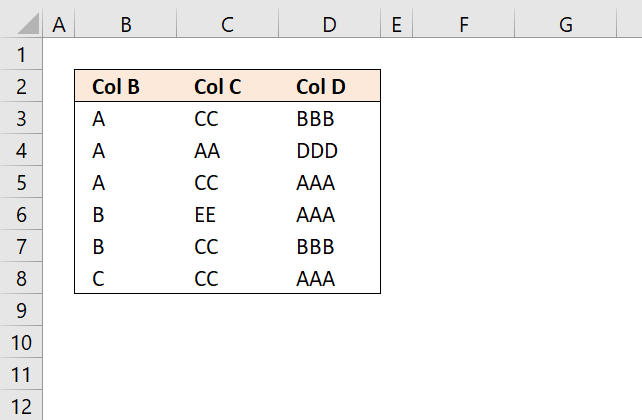You can also use Excel's Sort feature which is located on the "Home" tab on the ribbon.

The difference with this approach is that it is manual, you need to apply the following steps each time you add/delete or edit values contrary to the VBA macro and formulas.

1. Select the entire data set including the header names.
2. Go to tab "Home" on the ribbon.
3. Press with mouse on the "Sort & Filter" button.
4. Press with mouse on "Custom Sort..." and a dialog box appears.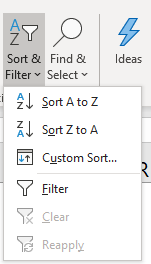5. Sort the first column by column B.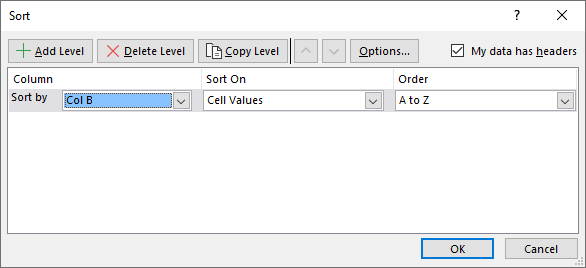6. Press with mouse on "Add Level" button.7. Sort the second column by column C.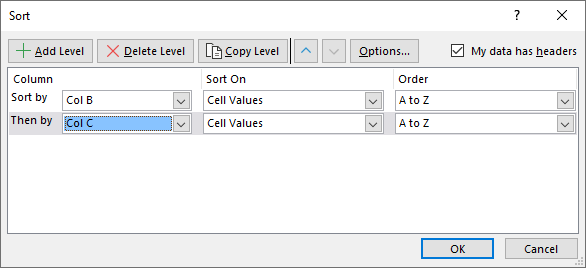8. Press with mouse on "Add Level" button.
9. Sort the third column by column D.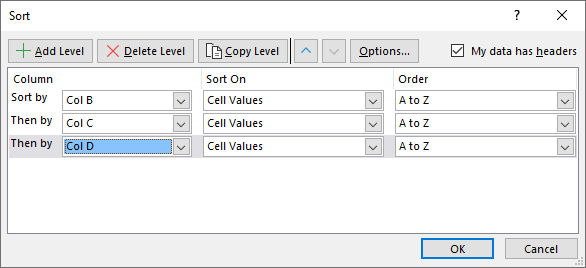10. Press with left mouse button on the OK button to apply changes.

Tip! Press with mouse on "A to Z" to change the sort order from "A to Z" to "Z to A". Disable checkbox "My data has headers" if your data set has no column names.

### Sort using VBA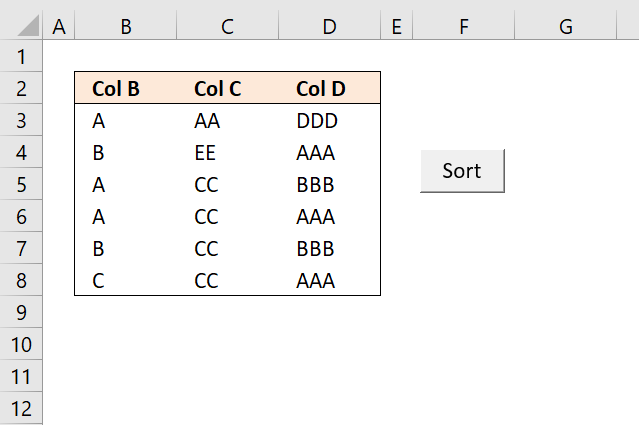This example demonstrates a button that is linked to a macro, press with left mouse button on the button to start the macro. I will also show you how to run the macro if a cell on the same worksheet has been changed using event code.

#### VBA code

```Sub Macro1()
'Allows you to write shorter code by referring to an object only once instead of using it with each property.
With ActiveWorkbook.Worksheets("VBA").Sort

'Clear previous sort conditions
.SortFields.Clear

'Apply sorting from A to Z to cell range B3:B8

'Apply sorting from A to Z to cell range C3:C8

'Apply sorting from A to Z to cell range D3:D8

'Set range to B2:D8
.SetRange Range("B2:D8")

'Column names exists

'No case sensitivity
.MatchCase = False

'Sort orientation xlTopToBottom vs xlLeftToRight
.Orientation = xlTopToBottom

'Apply sorting to range
.Apply
End With
End Sub
```

#### Where to put the VBA code?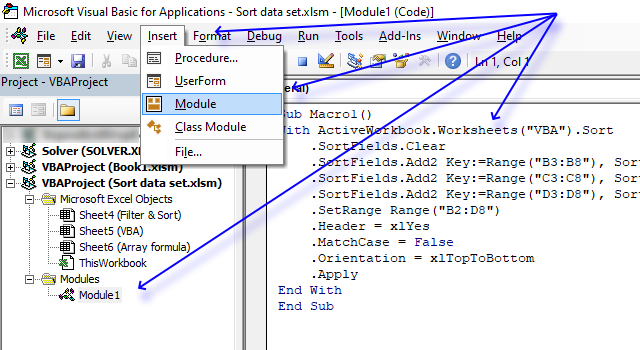1. Press Alt + F11 to open the Visual Basic Editor.
2. Press with mouse on "Insert" on the top menu.
3. Press with mouse on "Module" to insert a module to your workbook. It is named Module1 in the image above.
4. Copy above VBA code.
5. Paste to code window.
Note, save your workbook with file extension *.xlsm (macro-enabled workbook) to keep the code attached the workbook.

#### How to create a button linked to the macro1. Go to tab "Developer" on the ribbon.
2. Press with mouse on "Insert Controls" button.
3. Press with mouse on "Button" button.
4. Press with left mouse button on and drag on the worksheet to create the button. You can always later resize the button.
5. A dialog box appears asking for a macro to be assigned. Select the macro you want to use and press with left mouse button on OK.

#### Event code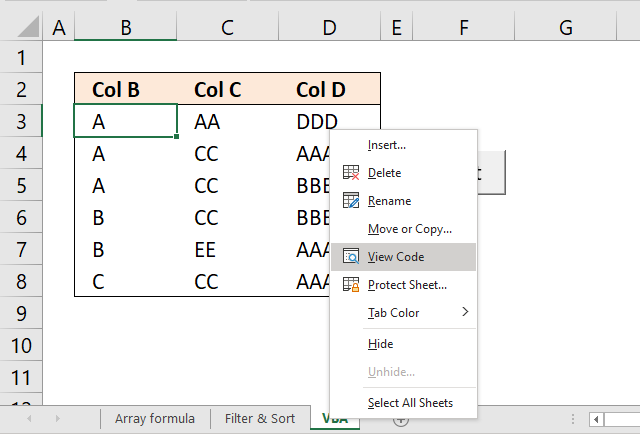Event code allows you to run the sort macro automatically based on specific things that can happen, for instance, activating the worksheet, selecting a cell or in this case, if a cell value has changed.

```'Event code that is rund when a cell value in worksheet VBA changes
Private Sub Worksheet_Change(ByVal Target As Range)

'Start macro named macro1
Macro1

End Sub
```

#### Where to put the Event code?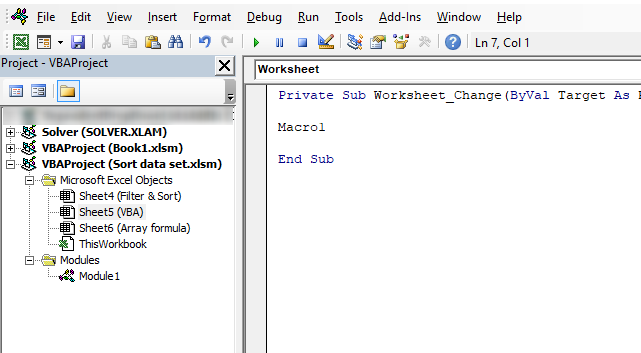1. Press with right mouse button on on the worksheet tab located at the very bottom of your Excel Screen.
2. Press with mouse on "View Code".3. The Visual Basic Editor opens with the worksheet module selected.
4. Copy and paste the event code above to the worksheet module.